Linear Algebra

Overview of the Course

The subject of this course is linear algebra.
Linear means the simplest kind of functions (after constants).
For example:
• f(x)=x
• f(x)=3x+1
• f(x)=-5x+1
• f(x)=1/2x-1

These are all linear.
BUT the following:
• f(x)=x^2
• f(x)=sin(x)
• f(x)=x^3-x
Are NOT linear.
The difference is more apparent if we draw graphs:

Linear: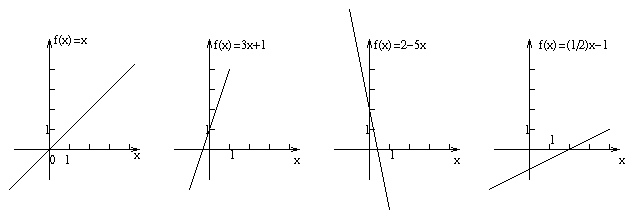Not Linear: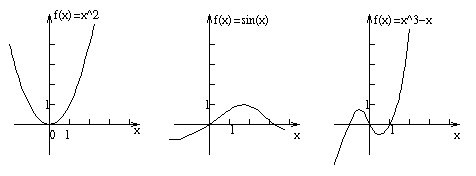The linear functions are simpler because they are just straight lines.
In a calculus course, you'll see more complicated functions, and use calculus to analyze them.
We're not going to look at anything more complicated than linear functions. But we are going to look at more than one at once, so things get complicated in a different way.

Eg.

What if I ask you to solve:
5x-2y=16
3x+4y=20

We could just solve it and stop there.
But there are several other ways of looking at the situation:
As a...
• Linear system
• Graph - use the geometry
• Augmented matrix (systemized method of solving.)
• Vector Equation
• Matrix Equation
• Transformation

Looking at something from a different point of view may give a new insight and enable us to solve the problem more easily.

The different ways of looking at the situation: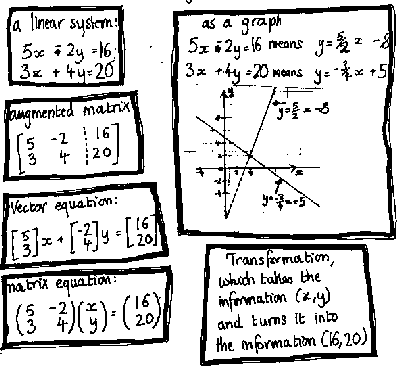In this course we're going to look at all the above ideas in some detail.

Outline of contents of lectures

We'll cover the following (not necessarily in exactly the following order). (I shall add corresponding section numbers in the book very soon).
Linear Systems
Definitions, simple examples, notation
The Geometry
How a solution can be represented by a point on a graph. Equations are lines. Lines can meet in different ways.
Transformations
How to think about a linear system as a transformation, and lots of other interesting transformations. Lots of pictures!
Vector spaces
Some interesting properties of solutions of linear systems. We'll see problems with many solutions. "vector spaces" is the name for a certain collection of solutions. We'll see how to get many solutions from a few "basic" solutions. We'll also study dimension. Eg., what is 3 about the 3-dimensional space what we live in? We'll also define inner product.
Vector Equations
Now we know about vector spaces, we'll look at linear systems as vector equations
Matrices
We'll go back and reformulate our ideas about linear systems and transformations in terms of a new notation: Matrices. But matrices are more than just a notation; we can do a lot of interesting things with them in their own right. We'll briefly look at how several other concepts can be formulated in terms of matrices.
Matrix Topics
• Transformations
• Determinant
• Rank
• Matrix multiplication
• Inverse of a matrix
• Eigen vectors, eigen values
• Diagonalization
Applications...
Finale
As a finale, we'll use the ideas in the course to look at some interesting mathematics (not decided what yet, maybe something to do with fractals...)(non examinable)

Linear Systems

First definitions and examples

Things like "x", "y", "z", stand for unknown quantities, and are called variables.
Things like "2", "3", "48072", "3.1415" are known, and are called constants.
Multiply a load of variables by a load of constants, add them up, and set them equal to a constant, and you get a linear equation.
Eg.
2x+3y+48072z=3.1415

And when we take a collection of linear equations, we get a linear system:
Eg.
x-2y+z=0
2y-8z=8
-4x+5y+9z=-9

(Note, variables can have all kinds of other names, eg, x1, x2, x3, x4, or r,s,t,u,v,w, etc.)

Example

"A mule and a donkey are struggling with a load of sacks. The donkey was groaning, so the mule said to him: 'Why to you complain? If you gave me one sack, I would have twice as many as you, and if I gave you one of my sacks, then we would be carrying equal loads.' How many did each have?"
(For practice, if you've seen this kind of thing before, you could think a few minutes about how to do this.)

Solution

Let D = number of sacks on the donkey's back
Let M = number of sacks on the Mule's back
Then:
M+1=2(D-1)=2D-2
M-1=D+1

So we get the following linear system:
M-2D=-3
M-D=2

Subtract the first from the second equation to get D=5. Then substitute the D=5 into the second equation to get M=7. (And check that it works as an answer to the original question!)
The solution is (D,M)=(5,7).

The Augmented Matrix and Row Operations

Another way of writing the above equation is: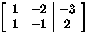We've put the linear system in an augmented matrix.
Now we call the addition or subtraction of different equations from each other row operations.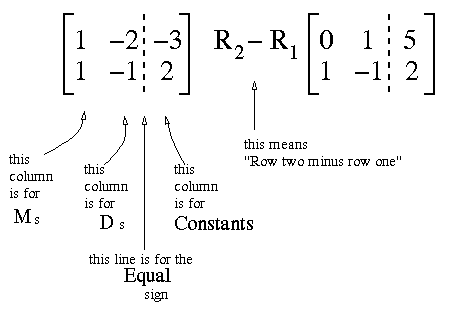Now, it might be obvious to us that from the first line of the augmented matrix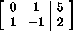we can find M, and then use that in the second line to find D.
But it might not be obvious to a computer. To systemize what we're doing, we put the equation to be solves first at the bottom: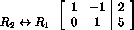(I've put a little note by the side of the matrix to indicate what I've done; swapped row 1 with row 2.)
The resulting augmented matrix is called in Echelon form.
On page 13 of the text book, there is a proper definition of Echelon form, and there is a list of exactly what the row operations are that you are allowed to do.
We'll look at a few more examples to motivate the definition first.

It's pretty easy to see how to solve the following set of equations:
 u + 2v + w - 3x - y + 2z = 3 v - 2w + 3x = 5 w - 2x - y + z = -4 x + 5y - 3z = 11 y + z = 11 z = 6

This easy, (though maybe a little tedious) to solve.
You start that the bottom and work up:
 z = 6 y = 11-x=11-6 = 5 x = 11+3z-5y=11+18-25 = 4

And so on. This is called Back substitution.
You should get (u,v,w,x,y,z)=(1,2,3,4,5,6).
The above is a lot easier to see what to do with than the following:
z=6
z+y=11
w+z-2x-y=-4
u+2v+2z-y-3x+w=3
-3z+5y+x=11
3x-2w+v=5

But actually, these are both the same sets of equations. So you can see how it helps to have them in a nice systematic order.

Echelon Form

Echelon form means that you out the equations so that the same variables are in each column, and each row is shorter than the previous, and starts after it. Echelon form is like the nice neat set of equations above, except you put the numbers into an augmented matrix: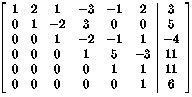Here's some more examples: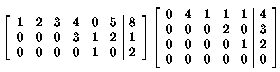See text book, page 13, for definition, and page 14 for more examples.

Reduced Echelon Form

Reduced Echelon form is even easier to solve. The first non zero entry of each row (the leading entry), is always 1, and above any leading entry, we get only zeros. For our example, the reduced Echelon form is: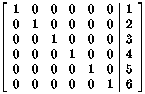Here are some other matrices in reduced echelon form: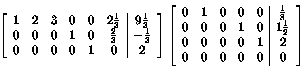To get to reduced Echelon form from Echelon form, you just start at the bottom and work up, just like back substitution.

Example of solving a linear system in reduced Echelon form

We'll show how to solve the first of the linear systems immediately above.
Make up some variables to correspond to the columns, eg, x1,x2,x3,x4,x5,x6.
Then from the bottom line of the matrix,
x5 = 2

But we can't determine x4 or x6 explicitly from the next line up. We just do the best we can, and write:
x4=-(1/3)-(2/3)x6

And from the top line of the matrix,
x1=28/3-7/3 x6 - 3 x3 -2 x2

If someone does supply us with additional information of what x6, x3, and x2 are, we can quickly find x5, x4, and x1.
We call x6, x3, x2 parameters of the system, or free variables.

How to get to Echelon form?

We've seen how to solve an equation in Echelon form, and in reduced Echelon form. And we saw for the Donkey and Mule example how to turn a linear system that was not in Echelon form into one that was. Now we need to know how to do this in general.
This is not very difficult, but it is fundamental, in the way that knowing multiplication tables is pretty fundamental in arithmetic. (And in the same way, people don't really have to do it - computers can be programmed to do the work.) Anyway, it's important to know how to solve a linear equation like this. Once that is done, we can get onto more interesting things.
We will look though some examples in class, starting with Example 1 on page 5 of the text book.

Homework

This is to be handed in to be marked on Tuesday 13th May.
• Read sections 1.1 and 1.2 of the text book
• Read sections 1.1 and 1.2 of the study guide
• From section 1.1, questions:
• 2
• 6
• 14
• 18
• 21
• 33
• From section 1.2, questions:
• 2
• 5
• 12
• 24
• 30
• For 1.1 Question 2, draw a graph of the given equations to find the solution.
• Make up a linear algebra problem, in words, with at least two variables, which has a unique solution. What is the solution? why is it unique?
• Make up a linear algebra problem, in words, with at least two variables, which has no solution. Why is there no solution?
• Make up a linear algebra problem, in words, with at least two variables, which has an infinite number of solutions. Describe the solutions.
• For each of the last three questions, if you used less than three variable, draw a graph of the initial equations used.

Solutions

Solutions to the assignment questions are now (from 22nd May) available on reserve from third floor of straufer library.

What will be on the Quiz

On Thursday 8th May, there will be a short quiz, with a question very similar to the following (just the numbers will be changed): Write the following linear system as an Augmented matrix, use row operations to get to reduced Echelon form. Write the solutions for x,y,z.
 2x + y + z = 8 x - y - z = -2 3x + y + 2z = 11

Solution

The augmented matrix is:
 / 2 1 1 | 8 \ | 1 -1 -1 | -2 | \ 3 1 2 | 11 /

(Note, those are supposed to be round brackets round the side.)
Next we do row reduction, the forward stage first, which means getting leading 1s with zeros underneath them.
Because there is a 1 in the second row of the first column, I'm going to swap second row with first row, as swapping is easier than dividing by 2.
 R1 <-> R2 / 1 -1 -1 | -2 \ | 2 1 1 | 8 | \ 3 1 2 | 11 /

(The place where this 1 is, in the first row, first column, is called a pivot)
Now take multiples of the first row from the second and third row, so that we eliminate the x from the corresponding equations.
First, row two minus 2 times row one.
 / 1 -1 -1 | -2 \ R2-2R1 | 0 3 3 | 12 | \ 3 1 2 | 11 /

Now deal with getting rid of the next non zero entry under the leading one. Do this by row three minus 3 times row 1.
 / 1 -1 -1 | -2 \ | 0 3 3 | 12 | R3-3R1 \ 0 4 5 | 17 /

Now the first column is in Echelon form. Next we look for the next leading non zero entry. We have a three in the second row, second column. Divide by three to get a one.
 / 1 -1 -1 | -2 \ R2/3 | 0 1 1 | 4 | \ 0 4 5 | 17 /

Now we want zeros under this pivot position. So take 4 times row two from row three.
 / 1 -1 -1 | -2 \ | 0 1 1 | 4 | R3-4R2 \ 0 0 1 | 1 /

This is the end of the forward stage. The matrix is now in Echelon form. The last row tells us that z=1. To get x and y, we can use back substitution. Back substitution is the backwards stage of row reduction, which will get us to reduced Echelon form.
Now we need to get zeros above the pivot positions, starting with the last pivot position.
So we take the last row from the second, and then add the last row to the first:
 / 1 -1 -1 | -2 \ R2-R3 | 0 1 0 | 3 | \ 0 0 1 | 1 /
 R1+R3 / 1 -1 0 | -1 \ | 0 1 0 | 3 | \ 0 0 1 | 1 /

Now the last pivot position is OK, zeros above (and nothing below). Next we deal with the second column:
 R1+R2 / 1 0 0 | 2 \ | 0 1 0 | 3 | \ 0 0 1 | 1 /
Now the matrix is in reduced Echelon form. The first column is for x, the second for y, and the third for z, so the matrix means:
 x = 2 y = 3 z = 1
So the solution is x=2, y=3, z=1.
Finally, it's a good idea to check this solution by substituting these values into the equations that we had in the first place (I know from experience how easy it is to make silly mistakes with arithmetic!) Check:
 2*2 + 3 + 1 = 8 2 - 3 - 1 = -2 3*2 + 3 + 2*1 = 11

We get the numbers we're supposed to have, so this must be the right solution.
Note, you don't need to put in all the explainations when you write a solution in a quiz, homework, or exam, (but make sure you understand them). Just write:

Solution without the explainations:

 / 2 1 1 | 8 \ | 1 -1 -1 | -2 | \ 3 1 2 | 11 / R1 <-> R2 / 1 -1 -1 | -2 \ | 2 1 1 | 8 | \ 3 1 2 | 11 / / 1 -1 -1 | -2 \ R2-2R1 | 0 3 3 | 12 | \ 3 1 2 | 11 / / 1 -1 -1 | -2 \ | 0 3 3 | 12 | R3-3R1 \ 0 4 5 | 17 / / 1 -1 -1 | -2 \ R2/3 | 0 1 1 | 4 | \ 0 4 5 | 17 / / 1 -1 -1 | -2 \ | 0 1 1 | 4 | R3-4R2 \ 0 0 1 | 1 / / 1 -1 -1 | -2 \ R2-R3 | 0 1 0 | 3 | \ 0 0 1 | 1 / R1+R3 / 1 -1 0 | -1 \ | 0 1 0 | 3 | \ 0 0 1 | 1 / R1+R2 / 1 0 0 | 2 \ | 0 1 0 | 3 | \ 0 0 1 | 1 /